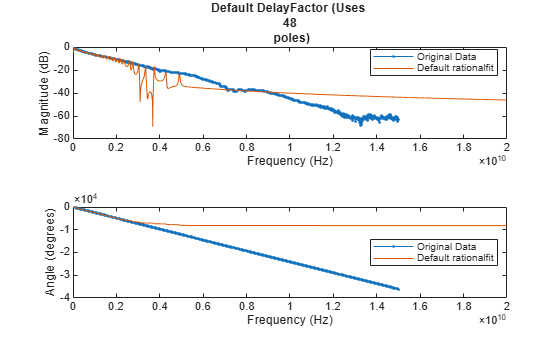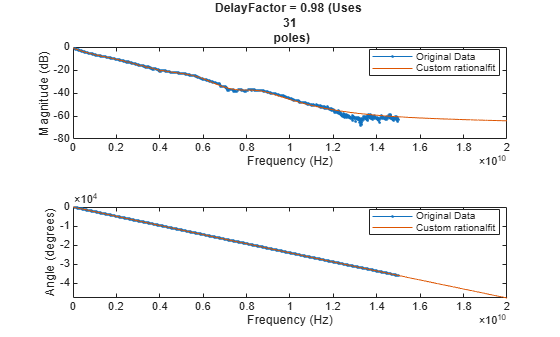# Using 'DelayFactor' Parameter With `rationalfit`

This example shows how to use the 'DelayFactor' parameter to improve the quality of the output of `rationalfit`.

The `rationalfit` function selects a rational function that matches frequency domain data. If that data contains a significant "time delay", which would present itself as a phase shift in the frequency domain, then it might be very difficult to fit using a reasonable number of poles.

In these cases, when the input data contains a large negative slope (i.e. data with a large enough time delay), we can ask `rationalfit` to first remove some of the delay from the data, and then find a rational function that best fits the remaining "undelayed" data. The `rationalfit` function accounts for the removed delay by storing it within the 'Delay' parameter of the output. By default, `rationalfit` does not remove any delay from the data.

First, create differential transfer function data from 4-port backplane S-parameters. Next, attempt to fit the data using the default settings of the `rationalfit` function. Lastly, use the 'DelayFactor' parameter to improve the accuracy of the output of `rationalfit`.

### Create Transfer Function

Read in the 4-port backplane S-parameter data from 'default.s4p'.

```S = sparameters('default.s4p'); fourportdata = S.Parameters; freq = S.Frequencies; fourportZ0 = S.Impedance;```

Convert 4-port single ended S-parameters into 2-port differential S-parameters

```diffdata = s2sdd(fourportdata); diffZ0 = 2*fourportZ0;```

Create a transfer function from the differential 2-port data

`tfdata = s2tf(diffdata,diffZ0,diffZ0,diffZ0);`

### Analyze Output of rationalfit When Using Default Value for 'DelayFactor'

Use the `freqresp` function to calculate the response of the output of `rationalfit`.

`defaultfit = rationalfit(freq,tfdata)`
```Warning: Achieved only -10.2 dB accuracy with 48 poles, not -40.0 dB. Consider specifying a larger number of poles using the 'NPoles' parameter. ```
```defaultfit = rfmodel.rational with properties: A: [48x1 double] C: [48x1 double] D: 0 Delay: 0 Name: 'Rational Function' ```
```respfreq = 0:4e6:20e9; defaultresp = freqresp(defaultfit,respfreq);```

Note that the 'Delay' parameter is zero (no delay removed from the data).

Plot the original data vs. the default output of `rationalfit`.

```figure subplot(2,1,1) tfdataDB = 20*log10(abs(tfdata)); plot(freq,tfdataDB,'.-') hold on plot(respfreq,20*log10(abs(defaultresp))) hold off xlabel('Frequency (Hz)') ylabel('Magnitude (dB)') defaultnpoles = numel(defaultfit.A); defstr = ['Default DelayFactor (Uses ',num2str(defaultnpoles),' poles)']; title(defstr) legend('Original Data','Default rationalfit','Location','best') subplot(2,1,2) tfdataphase = 180*unwrap(angle(tfdata))/pi; plot(freq,tfdataphase,'.-') hold on plot(respfreq,180*unwrap(angle(defaultresp))/pi) hold off xlabel('Frequency (Hz)') ylabel('Angle (degrees)') legend('Original Data','Default rationalfit','Location','best')```Note that the results when using the default settings of `rationalfit` are poor. Because the phase of the original data has a very large negative slope, it may be possible to improve the accuracy of the rational function by using the 'DelayFactor' parameter.

### Analyze Output of rationalfit When Using Custom Value for 'DelayFactor'

'DelayFactor' must be set to a value between 0 and 1. Choosing which value is an exercise in trial and error. For some data sets (those whose phase has an overall upward slope), changing the value of 'DelayFactor' will have no effect on the outcome.

Holding all other possible parameters of `rationalfit` constant, 0.98 is found to create a good fit.

`customfit = rationalfit(freq,tfdata,'DelayFactor',0.98)`
```customfit = rfmodel.rational with properties: A: [31x1 double] C: [31x1 double] D: 0 Delay: 6.5521e-09 Name: 'Rational Function' ```
`customresp = freqresp(customfit,respfreq);`

Note that the 'Delay' parameter is not zero (`rationalfit` removed some delay from the data).

Plot the original data vs. the custom output of `rationalfit`.

```subplot(2,1,1) plot(freq,tfdataDB,'.-') hold on plot(respfreq,20*log10(abs(customresp))) hold off xlabel('Frequency (Hz)') ylabel('Magnitude (dB)') customnpoles = numel(customfit.A); customstr = ['DelayFactor = 0.98 (Uses ',num2str(customnpoles),' poles)']; title(customstr) legend('Original Data','Custom rationalfit','Location','best') subplot(2,1,2) plot(freq,tfdataphase,'.-') hold on plot(respfreq,180*unwrap(angle(customresp))/pi) hold off xlabel('Frequency (Hz)') ylabel('Angle (degrees)') legend('Original Data','Custom rationalfit','Location','best')```The rational function created by using a custom value for 'DelayFactor' is much more accurate, and uses fewer poles.